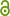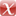# Backward error analysis for variational discretisations of partial differential equations

R.I. McLachlan, C. Offen, Journal of Geometric Mechanics 14 (2022) 447–471.Backward error analysis for variational discretisations of PDEs 1.51 MB
Journal Article | Published | English
Author
Department
Abstract
In backward error analysis, an approximate solution to an equation is compared to the exact solution to a nearby ‘modified’ equation. In numerical ordinary differential equations, the two agree up to any power of the step size. If the differential equation has a geometric property then the modified equation may share it. In this way, known properties of differential equations can be applied to the approximation. But for partial differential equations, the known modified equations are of higher order, limiting applicability of the theory. Therefore, we study symmetric solutions of discretized partial differential equations that arise from a discrete variational principle. These symmetric solutions obey infinite-dimensional functional equations. We show that these equations admit second-order modified equations which are Hamiltonian and also possess first-order Lagrangians in modified coordinates. The modified equation and its associated structures are computed explicitly for the case of rotating travelling waves in the nonlinear wave equation.
Publishing Year
Journal Title
Journal of Geometric Mechanics
Volume
14
Issue
3
Page
447 - 471
LibreCat-ID

### Cite this

McLachlan RI, Offen C. Backward error analysis for variational discretisations of partial  differential equations. Journal of Geometric Mechanics. 2022;14(3):447-471. doi:10.3934/jgm.2022014
McLachlan, R. I., & Offen, C. (2022). Backward error analysis for variational discretisations of partial  differential equations. Journal of Geometric Mechanics, 14(3), 447–471. https://doi.org/10.3934/jgm.2022014
@article{McLachlan_Offen_2022, title={Backward error analysis for variational discretisations of partial  differential equations}, volume={14}, DOI={10.3934/jgm.2022014}, number={3}, journal={Journal of Geometric Mechanics}, publisher={AIMS}, author={McLachlan, Robert I and Offen, Christian}, year={2022}, pages={447–471} }
McLachlan, Robert I, and Christian Offen. “Backward Error Analysis for Variational Discretisations of Partial  Differential Equations.” Journal of Geometric Mechanics 14, no. 3 (2022): 447–71. https://doi.org/10.3934/jgm.2022014.
R. I. McLachlan and C. Offen, “Backward error analysis for variational discretisations of partial  differential equations,” Journal of Geometric Mechanics, vol. 14, no. 3, pp. 447–471, 2022, doi: 10.3934/jgm.2022014.
McLachlan, Robert I., and Christian Offen. “Backward Error Analysis for Variational Discretisations of Partial  Differential Equations.” Journal of Geometric Mechanics, vol. 14, no. 3, AIMS, 2022, pp. 447–71, doi:10.3934/jgm.2022014.
All files available under the following license(s):
Main File(s)
File Name
File Title
Backward error analysis for variational discretisations of PDEs
Description
In backward error analysis, an approximate solution to an equa- tion is compared to the exact solution to a nearby ‘modified’ equation. In numerical ordinary differential equations, the two agree up to any power of the step size. If the differential equation has a geometric property then the modified equation may share it. In this way, known properties of differential equations can be applied to the approximation. But for partial differential equations, the known modified equations are of higher order, limiting appli- cability of the theory. Therefore, we study symmetric solutions of discretized partial differential equations that arise from a discrete variational principle. These symmetric solutions obey infinite-dimensional functional equations. We show that these equations admit second-order modified equations which are Hamiltonian and also possess first-order Lagrangians in modified coordinates. The modified equation and its associated structures are computed explicitly for the case of rotating travelling waves in the nonlinear wave equation.
Access LevelOpen Access
2022-06-13T09:11:38Z

Software:

### Export

Marked Publications

Open Data LibreCat

### SourcesarXiv 2006.14172